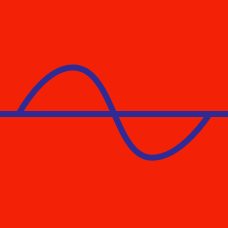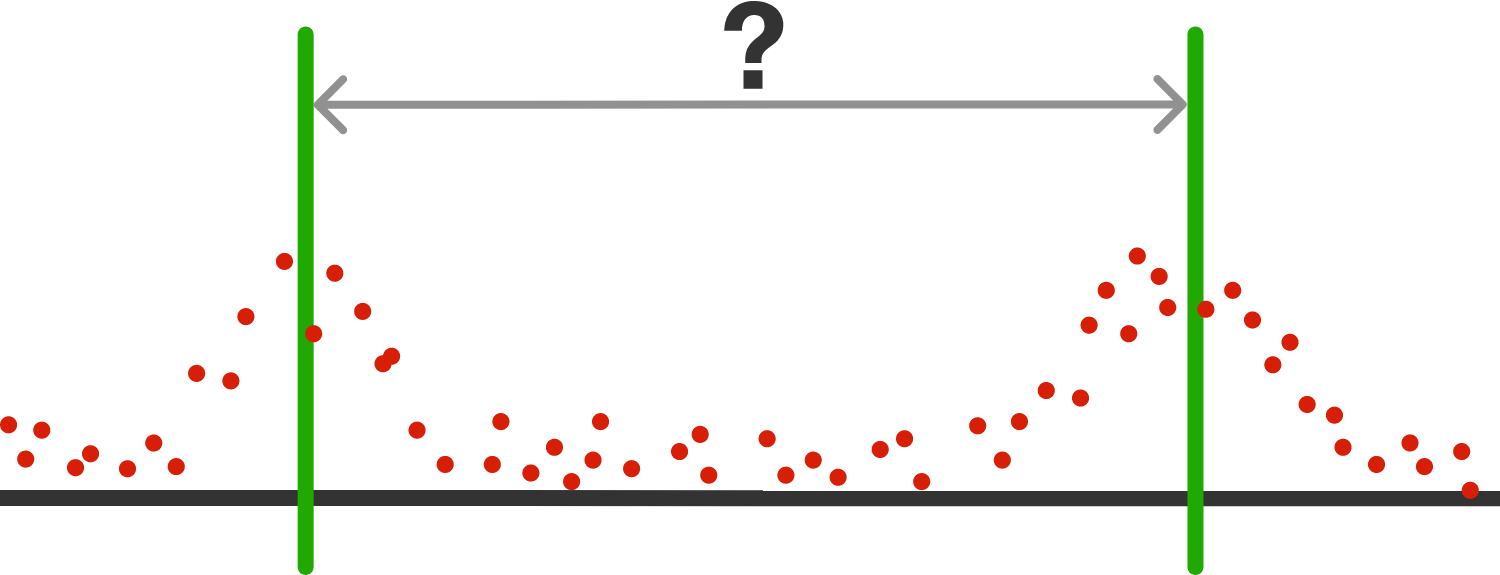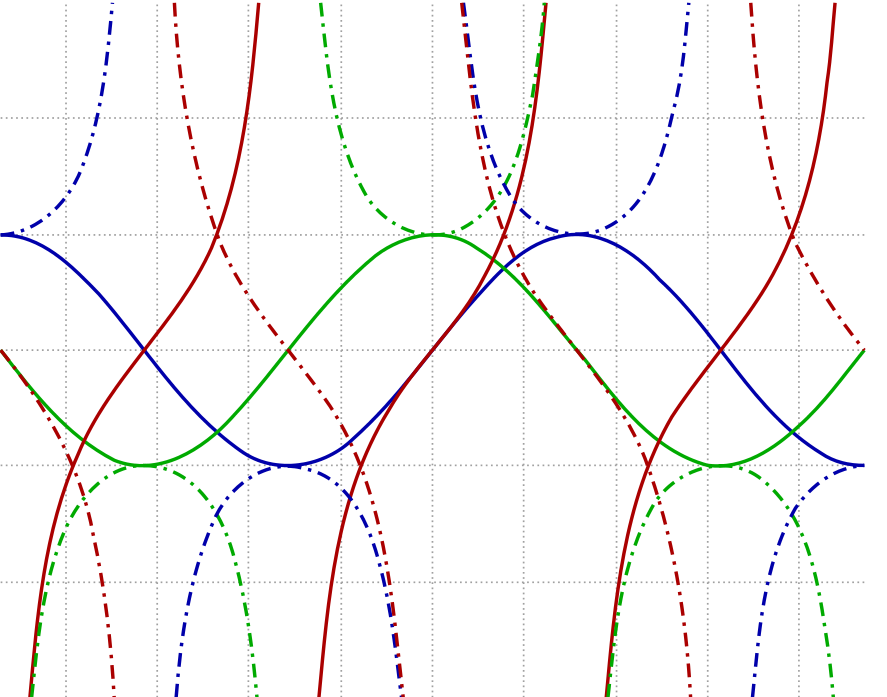Geometry

# Graphs of Trigonometric Functions: Level 3 Challenges$\large \color{orangered}{f(x)=\sin^6x+\cos^6x+\sin^4x \cos^2x + \cos^4x \sin^2x}$ Find the fundamental period of the function $\color{orangered}{f(x)}$.

Over the entire real line, the number of values of x where the function

$f(x) = \cos x + \cos ( \sqrt{2}x )$ attains its maximum value is:$\large \begin{cases}{y=\sin (x)} \\ {y=\cos (x)} \\ {y=\tan (x)} \\ {y=\csc (x)}\end{cases}$

The four graphs above are drawn on the same axes from $x = 0$ to $x = \frac \pi2$.

If a vertical line is drawn where the graphs of $y=\cos (x)$ and $y = \tan (x)$ intersect, this line intersects the graphs of $y=\sin(x)$ and $y=\csc(x)$ at points $A$ and $B$. What is the distance between $A$ and $B$?

How many real numbers $x$ satisfy $\sin x = \frac{x}{100}?$

What is the smallest positive integer $n$ such that $\large \displaystyle f(x)= \frac {\sin(\sin (nx))}{\tan \left(\frac{x}{n}\right) }$ has a fundamental period of $6\pi?$

×

Problem Loading...

Note Loading...

Set Loading...